Bert's blog

## More advanced sketch

Last week, I introduced sketch as a tool to generate 2D projections of truly 3D scenes that have the correct depth perception. The final result of that post was the drawing below: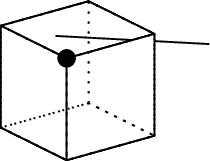This example was generated using the following sketch code:

def p000 (0, 0, 0)
def p001 (0, 0, 1)
def p010 (0, 1, 0)
def p011 (0, 1, 1)
def p100 (1, 0, 0)
def p101 (1, 0, 1)
def p110 (1, 1, 0)
def p111 (1, 1, 1)

def dpol0 polygon[lay=over,fill style=fill=none,line style=dotted]
(p000)(p010)(p011)(p001)
def dpol1 polygon[lay=over,fill style=fill=none,line style=dotted]
(p100)(p110)(p111)(p101)
def dpol2 polygon[lay=over,fill style=fill=none,line style=dotted]
(p000)(p001)(p101)(p100)
def dpol3 polygon[lay=over,fill style=fill=none,line style=dotted]
(p010)(p011)(p111)(p110)
def dpol4 polygon[lay=over,fill style=fill=none,line style=dotted]
(p000)(p100)(p110)(p010)
def dpol5 polygon[lay=over,fill style=fill=none,line style=dotted]
(p001)(p101)(p111)(p011)
def pol0 polygon(p000)(p010)(p011)(p001)
def pol1 polygon(p100)(p110)(p111)(p101)
def pol2 polygon(p000)(p001)(p101)(p100)
def pol3 polygon(p010)(p011)(p111)(p110)
def pol4 polygon(p000)(p100)(p110)(p010)
def pol5 polygon(p001)(p101)(p111)(p011)

def cube0 { {dpol0}{dpol1}{dpol2}{dpol3}{dpol4}{dpol5}
{pol0}{pol1}{pol2}{pol3}{pol4}{pol5} }

def line0 line(0.5, 0.5, -1)(1.5, 1.5, 1.5)

def dot0 dots(1, 1, 1)

def eye (20.0, 10.0, 15.)
def look_at (0.0, 0.0, 0.0)
put {view((eye), (look_at))} { {cube0}{line0}{dot0} }

global { language tikz }


In this week’s post, I want to expand on this by introducing some additional sketch features.

# Duplication and translation

One very neat feature of sketch is that it works incremental: if you assign an object to a variable (using def; the object itself can reference other objects stored in variables), then sketch simply stores the code that generates that object in that variable and literally pastes that into the final drawing command (the put in the cube example). Commands that are not part of an assignment are executed as they occur.

What this means is that we can very easily duplicate objects (like our example cube):

def cube1 put {translate([2.0, 0.0, 0.0])}{cube0}
put {view((eye), (look_at))} { {cube0}{cube1}{line0}{dot0} }


The last line replaces the original put command in the example. The resulting image looks like this: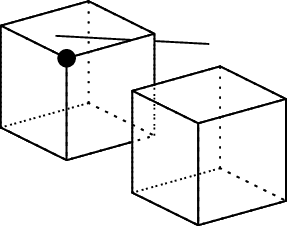Note that the original cube is now partially hidden by its copy, and that sketch correctly hides that part. The translate command is responsible for moving the copy of the cube, and takes a direction vector as argument. sketch distinguishes between positions (given in between round brackets - ()) and vectors (given in between square brackets - []). This distinction is important, as some operations (e.g. addition - +) are only permitted if both or at least one of the operands is a vector. This for geometrical reasons: adding two vectors or adding a vector to a position has geometrical meaning, while adding two positions does not have any geometrical meaning. Subtracting two positions on the other hand is allowed, as that geometrically results in a vector. This information can be quite useful when debugging weird error messages.

Apart from simple translations, sketch also supports rotations:

def cube1 put {rotate(45., (0.5, 0.5, 0.5), [0., 1., 0.])}{cube0}
def cube2 put {translate([2.0, 0.0, 0.0])}{cube1}
put {view((eye), (look_at))} { {cube0}{cube2}{line0}{dot0} }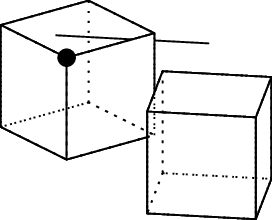And resizing:

def cube1 put {scale(1.6)}{cube0}
def cube2 put {translate([2.0, 0.0, 0.0])}{cube1}
put {view((eye), (look_at))} { {cube0}{cube2}{line0}{dot0} }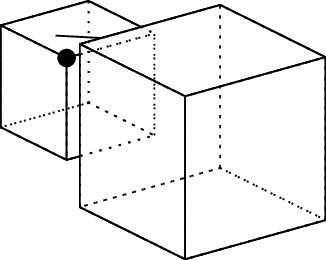# Specials

I already showed you how to change the line style for a line using the line style option (this is how we show the hidden edges of the cube). line style accepts many more options; all of these are directly passed on to the underlying TikZ draw command that sketch generates. The following would for example generate a red, dotted line:

def dpol2 polygon[lay=over,fill style=fill=none,line style=dotted,
line style=red]
(p000)(p001)(p101)(p100)


Clearly, having to add a line style or fill style for each individual TikZ option you want to add can become quite cumbersome, especially if you want to apply the same options to different objects. The solution is to use TikZ styles. These are usually defined in the preamble of the .tex wrapper document, like this:

\tikzstyle{dottedredline} = [dotted, red]


and can be used like this:

def dpol2 polygon[lay=over,fill style=fill=none,line style=dottedredline]
(p000)(p001)(p101)(p100)


This however has the annoying effect that it requires you to specify style options in two documents: the .sk file that contains the 3D scene, and the .tex document that just acts as a wrapper for the TikZ output that sketch generates. It clearly would be better if we could incorporate the \tikzstyle definition in the .sk file and keep everything together. To this end, we can use special:

special |\tikzstyle{dottedredline} = [dotted, red]|[lay=under]


special allows you to include anything in between || into the TikZ that sketch generates. Whether or not this makes sense depends on what you include; only valid TikZ or LaTeX commands will actually work when you compile the wrapper .tex file. Just like with normal objects, you can manipulate the position of the special object using the lay option. lay=under here means that the special will be put at the start of the output, and will hence definitely be defined when it is used as a TikZ option for any object.

You can also use special to add labels to a drawing:

def label0 { special |\node at #1 {\tiny{}label};|[lay=in](1, 0, 1) }
put {view((eye), (look_at))} { {cube0}{cube2}{line0}{dot0}{label0} }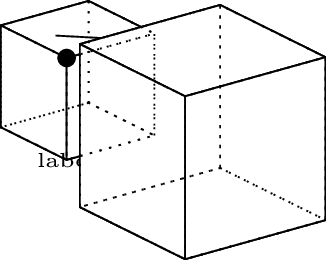There are a few things going on in this example: first of all, we added a \node and gave it the contents \tiny{}label, i.e. a string literal with one of the default LaTeX font sizes. This \node is positioned at the projected position #1, which corresponds to the position (1, 0, 1) that is passed on to the special object. To make sure the label gets the right depth perception as well, we use the lay=in option. This option only works if the first argument passed on to special is a position, and treats the entire special as if it was located on that position in the 3D drawing. Hence why part of the label is hidden in our example. Note the delimiting semicolon (;) in the special; this is required to generate valid TikZ.

Note that you can pass on as many arguments as you want to special, and that these can have different types (positions, vectors, scalars…). You can reference them using #1, #2.... 3D arguments are correctly transformed into their projected version before being passed on to the code in between ||. When lay=in is given, the first argument needs to be a position, and all contents of the special is treated as if it was at that location.

# Custom colours

As with any other draw/fill option, colours in sketch work the same as in TikZ, since it is ultimately the TikZ generated by sketch that gets converted into the final image. Which means that you have a choice of 19 predefined colours, or that you can try to appropriately mix these predefined colours using ! notation (as illustrated here).

Much easier is to define your own colours in the .tex preamble (using the xcolor support in TikZ):

\definecolor{winered}{RBG}{114, 47, 55}


This can also be wrapped in a special inside the .sk file:

special |\definecolor{winered}{RGB}{114, 47, 55}|[lay=under]


You can specify colours as shades of grey (gray), fractional RGB value (rgb), 8-bit RGB value (RGB), hexadecimal HTML format (HTML) or fractional CMYK values (cmyk), which should give you all the flexibility you would ever want.

# Putting it all together

The information contained in this post pretty much sums up my experience with sketch. sketch has many more powerful features, but I find that the features discussed here are sufficient for my needs. They have allowed me to create pretty interesting and powerful diagrams, including the cover image for this post: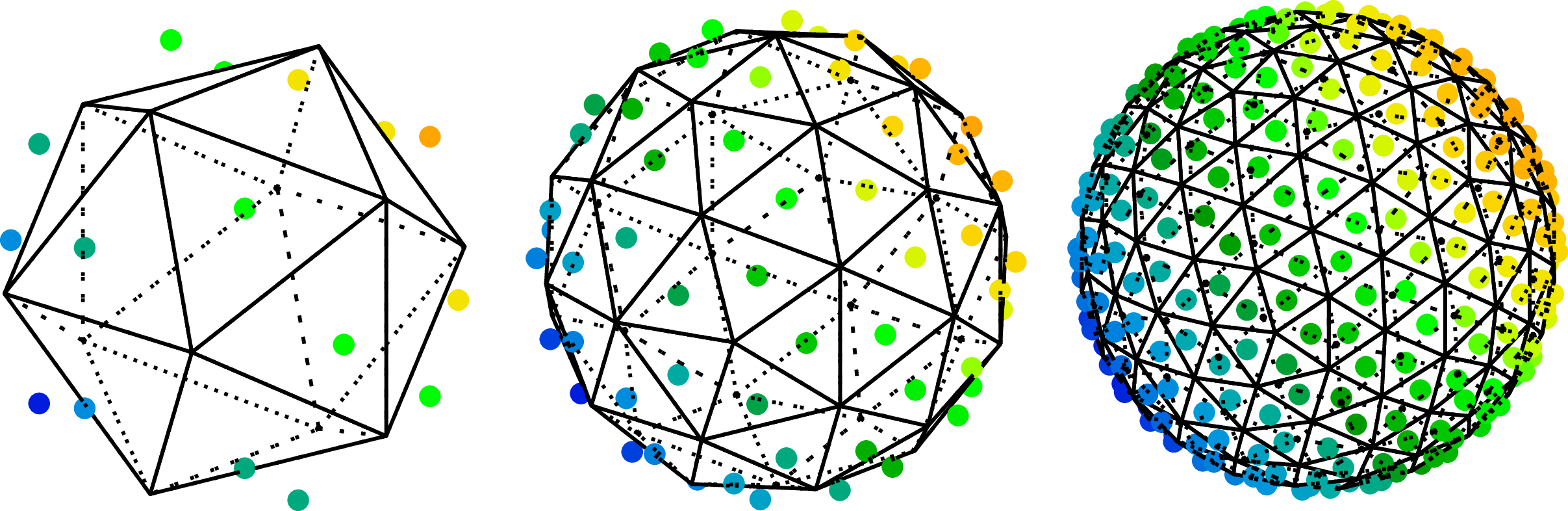The corresponding sketch.sk and sketch.tex source code files were generated using a Python script and only contain commands covered in this post.

Professional astronomer.